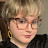This math video tutors and gives instruction about square and/or cube roots. The instruction is a brief and to the point teaching lesson on how to learn algebra and manipulate variables.
Square roots and cube roots are tricky to simplify and understand. This video is part of a series of videos that starts with simple problems and progresses to explain even the most difficult of problems that involve square roots and cube roots.

algebra tutors,algebra,college,cube,educational,equation,exponent,expression,for,ged,help,high,homework,how to,inequality,instruction,learn,lesson,linear,math,mathematics,online,practice,pre,property,pythagorean,root,school,simplify,solve,solving,square,system,teach,theorem,tutor,tutorial,tutoring,variable,video,with

algebratutors.org

1.Elaina Paige Wilkerson says: